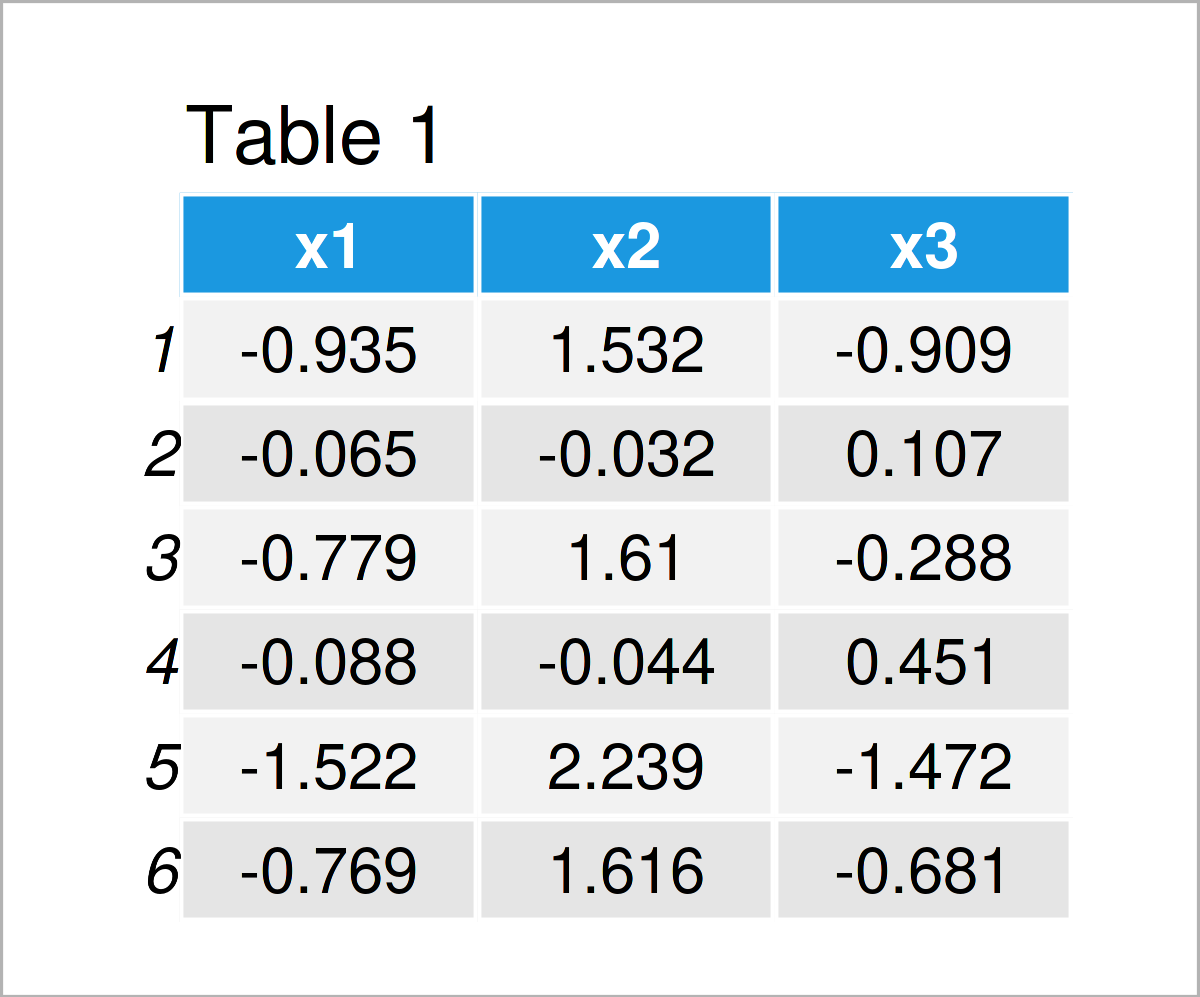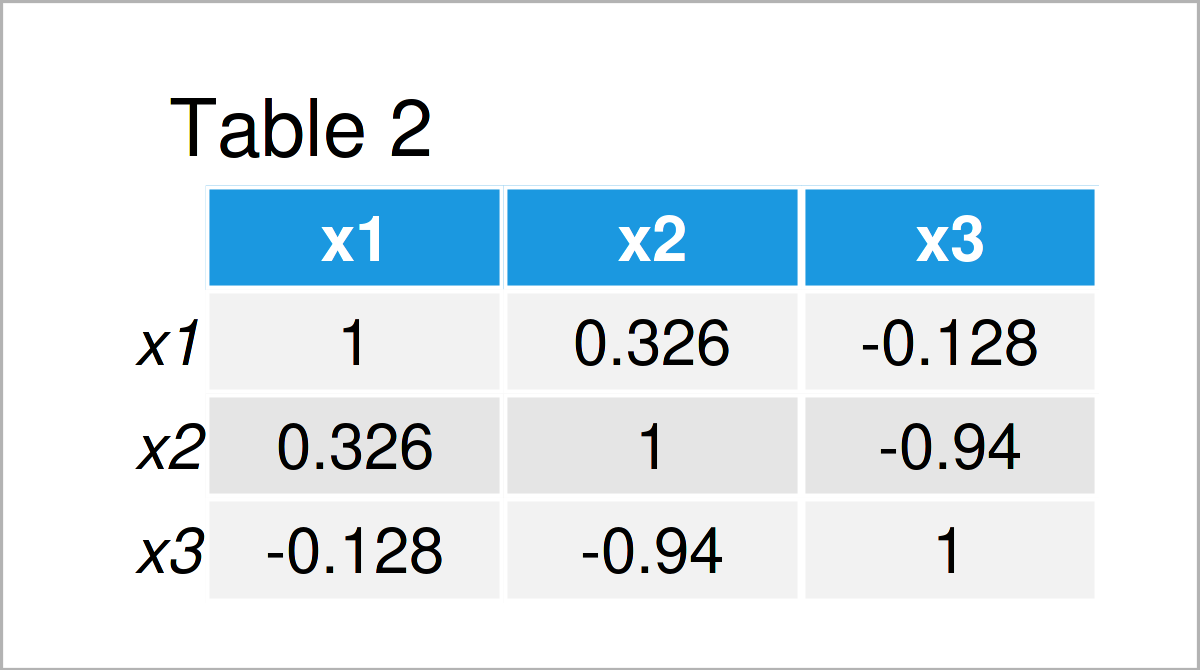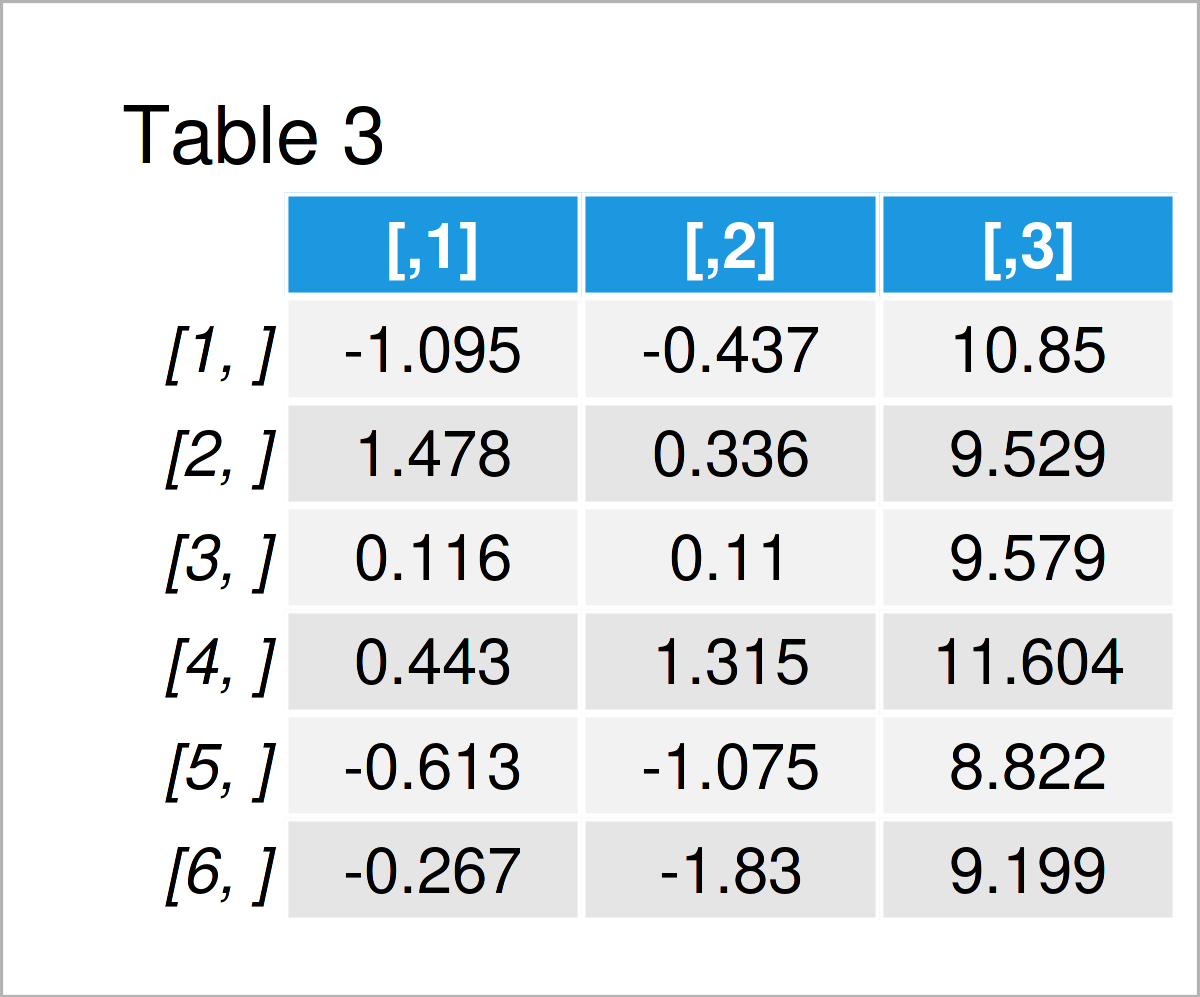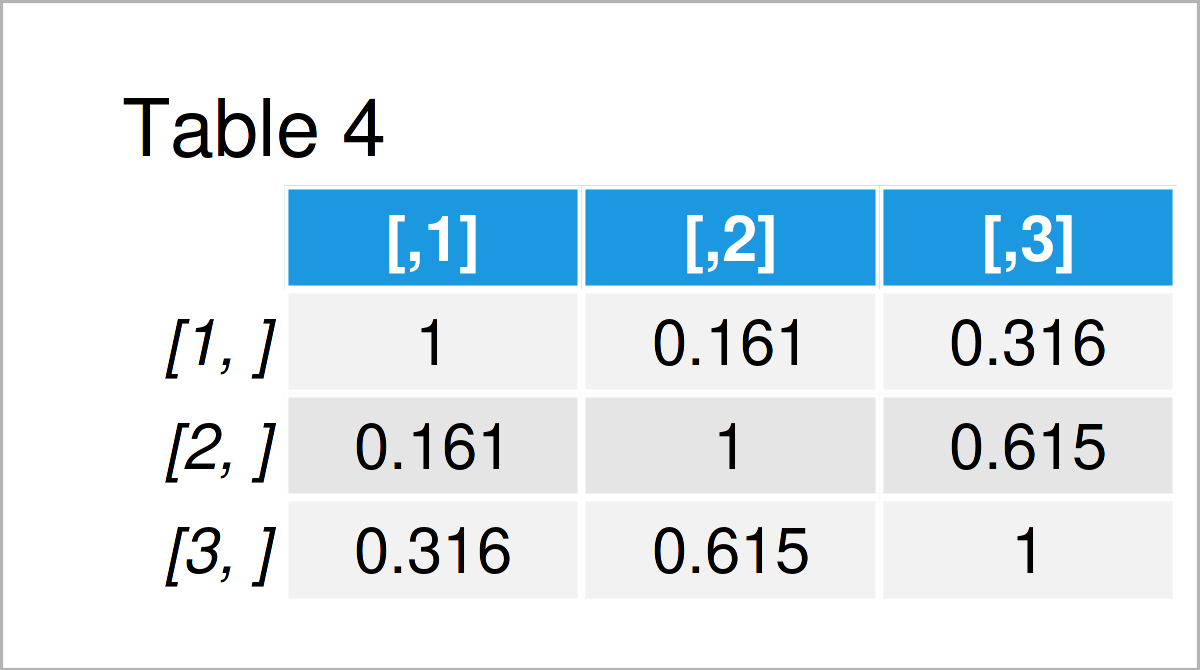# Generate Multivariate Random Data in R (2 Examples)

In this R article you’ll learn how to simulate multivariate random variables.

The article consists of the following:

Let’s just jump right in!

## Example 1: Generate Multivariate Random Data Manually

In Example 1, I’ll illustrate how to simulate multivariate random data frame columns using the basic features of the R programming language.

As very first step, we should set a random seed for reproducibility of our code:

`set.seed(354627)                  # Set random seed`

In the next step, we can use random data generating functions such as rnorm (normal distribution), rpois (Poisson distribution), and runif (uniform distribution) to create a random data set:

```x1 <- rnorm(1000)                 # Create random data
x2 <- rpois(1000, 2) + 0.5 * x1
x3 <- runif(1000) + 0.2 * x1 - 0.7 * x2
data1 <- data.frame(x1, x2, x3)After running the previous R syntax the randomly drawn data frame shown in Table 1 has been created.

Note that we have added a fraction of some of the variables to the output of the random number generating functions. This ensures that our variables are correlated, as you can see by calculating the correlation matrix for our random data:

`cor(data1)                        # Correlation matrix of random data`As shown in Table 2, the columns of our random data set are correlated.

## Example 2: Generate Multivariate Random Data Using mvrnorm() Function of MASS Package

Even though the code of Example 1 worked fine, it is relatively complicated.

In Example 2, I’ll therefore demonstrate how to draw multivariate random numbers using the mvrnorm function of the MASS package.

We first have to install and load the MASS package:

```install.packages("MASS")          # Install MASS package

In the next step, we can use the mvrnorm function to draw normally distributed random numbers. In the following syntax, the n argument specifies the sample size, the mu argument specifies the mean values of each column, and the Sigma argument specifies the correlation matrix of our data:

```data2 <- mvrnorm(n = 1000,        # Create random data
mu = c(0.5, 0, 10),
Sigma = matrix(c(1, 0.2, 0.3,
0.2, 1, 0.6,
0.3, 0.6, 1),
nrow = 3))As shown in Table 3, the previous R programming code has constructed another random data set with three variables.

Let’s have a look at the correlation matrix of our data:

`cor(data2)                        # Correlation matrix of random data`As shown in Table 4, the correlations of our random data are approximately following the correlations we have specified within the mvrnorm function.

## Video & Further Resources

Do you need further info on the R programming code of this post? Then you might want to watch the following video that I have published on my YouTube channel. I’m explaining the examples of this tutorial in the video.

Please accept YouTube cookies to play this video. By accepting you will be accessing content from YouTube, a service provided by an external third party.If you accept this notice, your choice will be saved and the page will refresh.

In addition, you may read the related R tutorials on my website. A selection of related articles that are related to the simulation of multivariate random variables is shown below:

This tutorial has demonstrated how to simulate multivariate random data in R. In case you have further questions, don’t hesitate to let me know in the comments below. Furthermore, don’t forget to subscribe to my email newsletter to receive regular updates on the newest tutorials.

Subscribe to the Statistics Globe Newsletter

•Iguodala Edwin
May 5, 2023 6:32 am

Good morning sir, please I need help on how to generate multivariate normal random data set for higher dimensional data, such as n=50 p=100, q=150. this is to enable me run a CCA with my model

•May 8, 2023 9:35 am

Hello Iguodala,

Would this solution work for you? I assumed that p and q are the number of variables of two datasets and n is the number of observations in each dataset. If its wrong, you can adapt the given code accordingly.

```# Set your dimensions
n <- 50
p <- 100
q <- 150

# Create custom mean vectors for the p and q dimensions
mean_p <- rnorm(p, mean=0, sd=1)
mean_q <- rnorm(q, mean=0, sd=1)

# Create custom covariance matrices for the p and q dimensions
cov_p <- matrix(rnorm(p^2, mean=0, sd=1), nrow=p, ncol=p)
cov_p <- cov_p %*% t(cov_p)  # Make it symmetric and positive semi-definite
cov_q <- matrix(rnorm(q^2, mean=0, sd=1), nrow=q, ncol=q)
cov_q <- cov_q %*% t(cov_q)  # Make it symmetric and positive semi-definite

# Generate the multivariate normal random data sets
data_p <- mvrnorm(n, mean_p, cov_p)
data_q <- mvrnorm(n, mean_q, cov_q)```

Regards,
Cansu